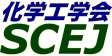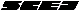## \$B9V1i%W%m%0%i%`!J2q>l!&F|DxJL!K(B

### A\$B2q>l(B \$BBh(B2\$BF|(B

\$B:G=*99?7F|;~!'(B2012-08-20 15:02:17
\$B9V1i(B
\$B;~9o(B
\$B9V1i(B
\$BHV9f(B
\$B9V1iBjL\!?H/I=\$B%-!<%o!<%I(B\$BJ,N`(B
\$BHV9f(B
\$B\$BHV9f(B
\$B%7%s%]%8%&%`(B \$B!c%P%\$%*\$K\$h\$k<+A3%(%M%k%.!
(13:20\$B!A(B14:00)\$B!!(B(\$B:BD9(B \$B7'C+(B \$BAo(B)
13:20\$B!A(B 13:40A214\$B23
(\$B4tIlBg1!9)(B) \$B!{(B(\$B3X(B)\$BI~It(B \$B9R4u(B \$B!&(B (\$B@5(B)\$B7(It(B \$BOB90(B \$B!&(B (\$B@5(B)\$B \$B!&(B (\$B3X(B)\$B6L0f(B \$BfF(B \$B!&(B (\$B@5(B)\$B?@86(B \$B?.;V(B
sewage sludge char
gas cleaning
ammonium sulfate
S-14200
13:40\$B!A(B 14:00A215\$B%?!<%k!&%A%c!<@\?(\$H%+%j%&%`?(G^:nMQ6/2=\$K\$h\$kDc;@AGHf!&Dc?e>x5\$Hf%P%\$%*%^%9%,%92=(B
(\$B6eBg@hF38&(B) \$B!{(B(\$B3X(B)\$BHxCS(B \$BCR9,(B \$B!&(B (\$B@5(B)\$B9)F#(B \$B??Fs(B \$B!&(B (\$B@5(B)\$BB'1J(B \$B9TMG(B \$B!&(B (\$B@5(B)\$BNS(B \$B=a0lO:(B
Biomass
Gasification
S-14721
(14:00\$B!A(B15:00)\$B!!(B(\$B:BD9(B \$BNS(B \$B=a0lO:(B)
14:00\$B!A(B 14:20A216Thermal Compression on Hybrid Poplar Wood: Polysaccharides Analysis
(UTP) \$B!{(B(\$B@5(B)Osman Noridah \$B!&(B (UI) McDonald Gabriel \$B!&(B (UoF) Laborie Marie-Pierre
Wood polysaccharide
thermal compression
FT-IR and FT-Raman
S-14610
14:20\$B!A(B 14:40A217(\$B9V1iCf;_(B)

100728
14:40\$B!A(B 15:00A218\$B
(\$B6eBgC:AG;q8;(B) \$B!{(B(\$B@5(B)\$B7'C+(B \$BAo(B \$B!&(B (\$B6eBg9)(B) \$BLnCf(B \$BATBY(B \$B!&(B \$BJ?Eg(B \$B9d(B
hydrothermal carbonization
bamboo
solid fuel
S-14950

\$B9V1i%W%m%0%i%`(B
\$B2=3X9)3X2q(B \$BBh(B44\$B2s=)5(Bg2q(B(C) 2012 \$B8x1W
Most recent update: 2012-08-20 15:02:17
For more information contact \$B2=3X9)3X2qElKL;YIt(B \$BBh(B44\$B2s=)5(Bg2q(B \$BLd\$\$9g\$;78(B
E-mail: inquiry-44fwww3.scej.org
This page was generated byeasp 2.28; proghtml 2.28c (C)1999-2011 kawase